Deviations from the Ideal Gas Law

Deviations from Ideal Gas Law Behavior

Van der Waals Equation

The behavior of real gases usually agrees with the predictions of the ideal gas equation to within5% at normal temperatures and pressures. At low temperatures or high pressures, real gases deviate significantly from ideal gas behavior. In 1873, while searching for a way to link the behavior of liquids and gases, the Dutch physicist Johannes van der Waals developed an explanation for these deviations and an equation that was able to fit the behavior of real gases over a much wider range of pressures.

Van der Waals realized that two of the assumptions of the kinetic molecular theory were questionable. The kinetic theory assumes that gas particles occupy a negligible fraction of the total volume of the gas. It also assumes that the force of attraction between gas molecules is zero.

The first assumption works at pressures close to 1 atm. But something happens to the validity of this assumption as the gas is compressed. Imagine for the moment that the atoms or molecules in a gas were all clustered in one corner of a cylinder, as shown in the figure below. At normal pressures, the volume occupied by these particles is a negligibly small fraction of the total volume of the gas. But at high pressures, this is no longer true. As a result, real gases are not as compressible at high pressures as an ideal gas. The volume of a real gas is therefore larger than expected from the ideal gas equation at high pressures.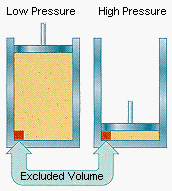Van der Waals proposed that we correct for the fact that the volume of a real gas is too large at high pressures by subtracting a term from the volume of the real gas before we substitute it into the ideal gas equation. He therefore introduced a constant

constant (b) into the ideal gas equation that was equal to the volume actually occupied by a mole of gas particles. Because the volume of the gas particles depends on the number of moles of gas in the container, the term that is subtracted from the real volume of the gas is equal to the number of moles of gas times b.

P(V - nb) = nRT

When the pressure is relatively small, and the volume is reasonably large, the nb term is too small to make any difference in the calculation. But at high pressures, when the volume of the gas is small, the nb term corrects for the fact that the volume of a real gas is larger than expected from the ideal gas equation.

The assumption that there is no force of attraction between gas particles cannot be true. If it was, gases would never condense to form liquids. In reality, there is a small force of attraction between gas molecules that tends to hold the molecules together. This force of attraction has two consequences: (1) gases condense to form liquids at low temperatures and (2) the pressure of a real gas is sometimes smaller than expected for an ideal gas.

To correct for the fact that the pressure of a real gas is smaller than expected from the ideal gas equation, van der Waals added a term to the pressure in this equation. This term contained a second constant (a) and has the form: an2/V2. The complete van der Waals equation is therefore written as follows.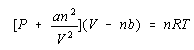This equation is something of a mixed blessing. It provides a much better fit with the behavior of a real gas than the ideal gas equation. But it does this at the cost of a loss in generality. The ideal gas equation is equally valid for any gas, whereas the van der Waals equation contains a pair of constants (a and b) that change from gas to gas.

The ideal gas equation predicts that a plot of PV versus P for a gas would be a horizontal line because PV should be a constant. Experimental data for PV versus P for H2 and N2 gas at 0C and CO2 at 40C are given in the figure below. Values of the van der Waals constants for these and other gases are given in the table below.

van der Waals Constants for Various Gases

 Compound a (L2-atm/mol2) b (L/mol) He 0.03412 0.02370 Ne 0.2107 0.01709 H2 0.2444 0.02661 Ar 1.345 0.03219 O2 1.360 0.03803 N2 1.390 0.03913 CO 1.485 0.03985 CH4 2.253 0.04278 CO2 3.592 0.04267 NH3 4.170 0.03707

The magnitude of the deviations from ideal gas behavior can be illustrated by comparing the results of calculations using the ideal gas equation and the van der Waals equation for 1.00 mole of CO2 at 0oC in containers of different volumes. Let's start with a 22.4 L container.

According to the ideal gas equation, the pressure of this gas should be 1.00 atm.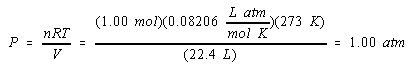Substituting what we know about CO2 into the van der Waals equation gives a much more complex equation.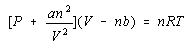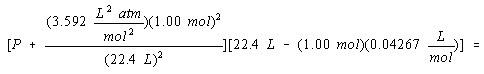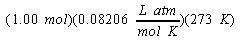This equation can be solved, however, for the pressure of the gas.

P = 0.995 atm

At normal temperatures and pressures, the ideal gas and van der Waals equations give essentially the same results.

Let's now repeat this calculation, assuming that the gas is compressed so that it fills a container that has a volume of only 0.200 liters. According to the ideal gas equation, the pressure would have to be increased to 112 atm to compress 1.00 mol of CO2 at 0C to a volume of 0.200 L.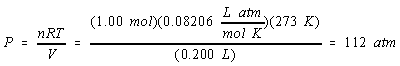The van der Waals equation, however, predicts that the pressure will only have to increase to 52.6 atm to achieve the same results.P = 52.6 atm

As the pressure of CO2 increases the van der Waals equation initially gives pressures that are smaller than the ideal gas equation, as shown in the figure below, because of the strong force of attraction between CO2 molecules.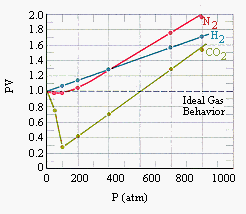A plot of the product of the pressure times the volume for samples of H2, N2, CO2 gases versus the pressure of these gases.

Let's now compress the gas even further, raising the pressure until the volume of the gas is only 0.0500 liters. The ideal gas equation predicts that the pressure would have to increase to 448 atm to condense 1.00 mole of CO2 at 0oC to a volume of 0.0500 L.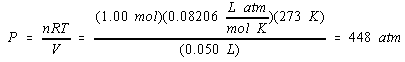The van der Waals equation predicts that the pressure will have to reach 1620 atm to achieve the same results.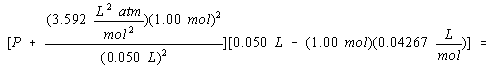P = 1620 atm

The van der Waals equation gives results that are larger than the ideal gas equation at very high pressures, as shown in the figure above, because of the volume occupied by the CO2 molecules.Analysis of the van der Waals Constants

The van der Waals equation contains two constants, a and b, that are characteristic properties of a particular gas. The first of these constants corrects for the force of attraction between gas particles. Compounds for which the force of attraction between particles is strong have large values for a. If you think about what happens when a liquid boils, you might expect that compounds with large values of a would have higher boiling points. (As the force of attraction between gas particles becomes stronger, we have to go to higher temperatures before we can break the bonds between the molecules in the liquid to form a gas.) It isn't surprising to find a correlation between the value of the a constant in the van der Waals equation and the boiling points of a number of simple compounds, as shown in the fugure below. Gases with very small values of a, such as H2 and He, must be cooled to almost absolute zero before they condense to form a liquid.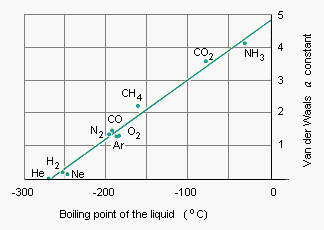The other van der Waals constant, b, is a rough measure of the size of a gas particle. According to the table of van der Waals constants, the volume of a mole of argon atoms is 0.03219 liters. This number can be used to estimate the volume of an individual argon atom.The volume of an argon atom can then be converted into cubic centimeters using the appropriate unit factors.If we assume that argon atoms are spherical, we can estimate the radius of these atoms. We start by noting that the volume of a sphere is related to its radius by the following formula.

V = 4/3 r3

We then assume that the volume of an argon atom is 5.345 x 10-23 cm and calculate the radius of the atom.

r = 2.3 x 10-8 cm

According to this calculation, an argon atom has a radius of about 2 x 10-8 cm.